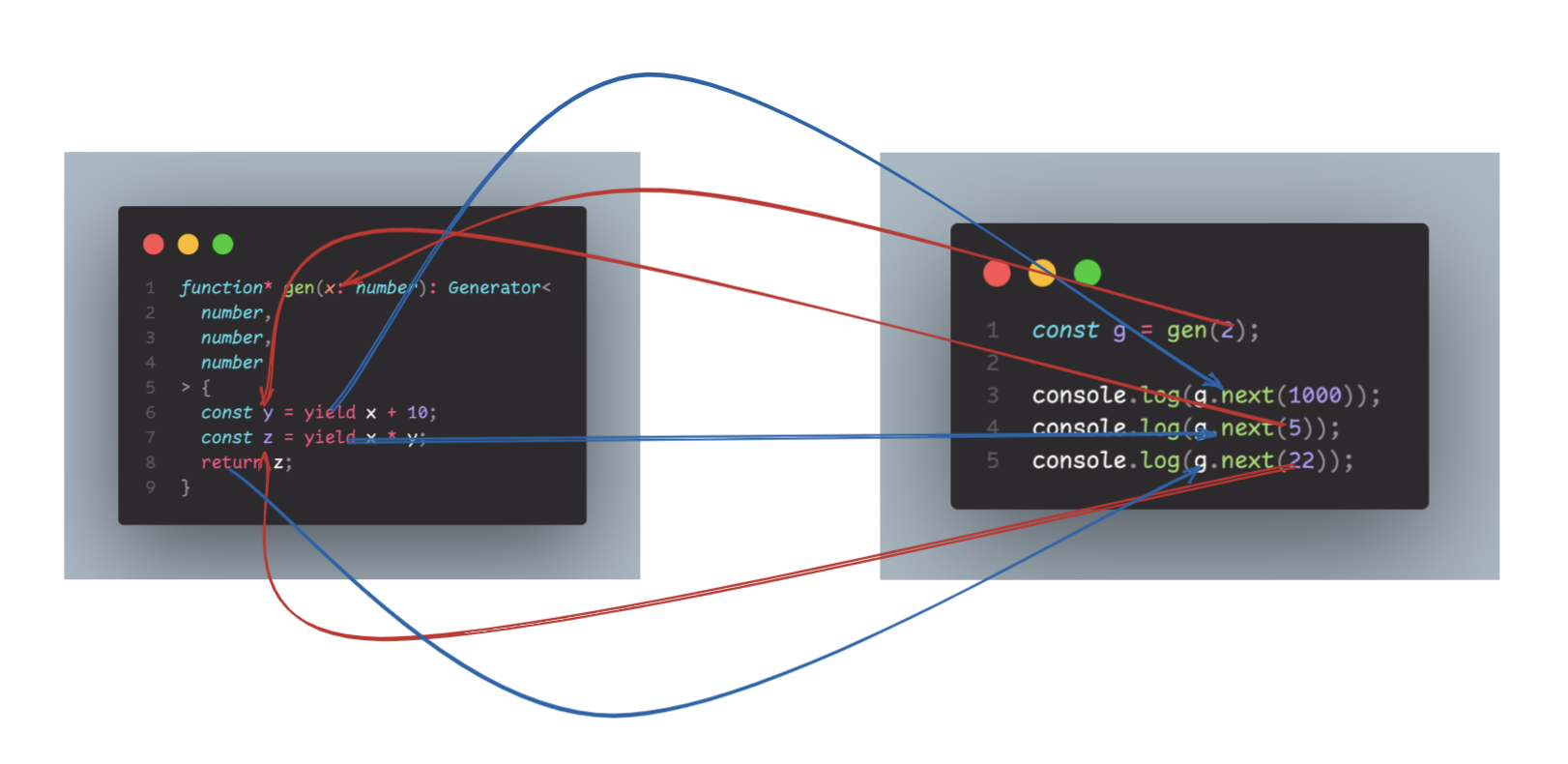### 语法

function* name([param[, param[, ... param]]]) { statements }

• name：函数名
• param：要传递给函数的一个参数的名称，一个函数最多可以有 255 个参数。
• statements：普通 JS 语句。

function* gen(x: number): Generator<
number,
number,
number
> {
const y = yield x + 10;
const z = yield x * y;
return z;
}

const g = gen(2);

console.log(g.next(1000)); // { value: 12, done: false }
console.log(g.next(5)); // { value: 10, done: false }
console.log(g.next(22)); // { value: 22, done: true }


interface Generator<
T = unknown,
TReturn = any,
TNext = unknown
> extends Iterator<T, TReturn, TNext> {
// NOTE: 'next' is defined using a tuple to ensure
// we report the correct assignability errors in all places.
next(...args: [] | [TNext]): IteratorResult<T, TReturn>;
return(value: TReturn): IteratorResult<T, TReturn>;
throw(e: any): IteratorResult<T, TReturn>;
[Symbol.iterator](): Generator<T, TReturn, TNext>;
}function* anotherGenerator(i: number) {
yield i + 1;
yield i + 2;
yield i + 3;
}

function* generator(i: number){
yield i;
yield* anotherGenerator(i);// 移交执行权
yield i + 10;
}

var gen = generator(10);

console.log(gen.next().value); // 10
console.log(gen.next().value); // 11
console.log(gen.next().value); // 12
console.log(gen.next().value); // 13
console.log(gen.next().value); // 20


### 随堂作业

function* iterArr(arr) {
if (Array.isArray(arr)) {
for (let i = 0; i < arr.length; i++) {
yield* iterArr(arr[i]);
}
} else {
yield arr;
}
}

var arr = ['a', ['b', 'c'], ['d', 'e']];
for (var x of iterArr(arr)) {
console.log(x);
}

var arr = ['a', ['b', ['c', ['d', 'e']]]];
var gen = iterArr(arr);
arr = [...gen];### 拓展

function req1() {
return new Promise((resolve) => {
setTimeout(() => resolve(2), 1000)
})
}

function req2() {
return new Promise((resolve) => {
setTimeout(() => resolve(22), 2000)
})
}

async function exec() {
const res1 = await req1();
const res2 = await req2();
return res1 + res2;
}

exec().then(console.log) // 22


const co = require('co');

function* exec() {
const res1 = yield req1()
const res2 = yield req2()
return res1 + res2
}

co(exec).then(console.log) // 22


Generator 函数就是一个异步操作的容器。它的自动执行需要一种机制，当异步操作有了结果，能够自动交回执行权。

co 函数库其实就是将两种自动执行器（Thunk 函数和 Promise 对象），包装成一个库。

••